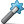#GMDH Shell Documentation

### Sidebar

#### Reference

one-click_examples

## One-Click Examples

GMDH Shell has several built-in example projects that can be recalculated in just one click at the Start button. You can load one of the examples using the Wizard dialog.

### Airline passengers

This is an example of time series forecasting. Original source of data is a book by `Box and Jenkins (1976): Times Series Analysis: Forecasting and Control, p. 531.` The dataset consists of 144 monthly totals of airline passengers from January 1949 to December 1960.

In this example project we generate one-year-ahead forecast for 12 latest observations as if we don't know them. We hold-out 12 latest values of output variable and create a model using the rest of data. In this way we can compare the result to actual data. In the Performance panel Mean absolute percentage error (MAPE) calculated for 12 predictions is 1.95% (percentage of target values).

### TOP500 supercomputers

This is an example of time series forecasting. Data source is `The Top500 List of Supercomputer Sites` released semiannually by www.top500.org. Every list contains information about different parameters of modern supercomputers including rank of a computer system, theoretical and measured computational power, the number of processing cores, manufacturer information, component types and models, operating system, etc. Example data is a historical list of entry level supercomputers from Jun 1993 to Nov 2008.

The task is to predict the entry-level supercomputer performance for the next Top500 release (Jun 2009). Since the Jun 2009 is in the past, we know that the entry-level performance was 17088 Gflop/s.

In order to estimate future accuracy of our prediction for the unknown Jun 2009, we've checked how accurate would be predictions of 3 time-points in the past if we would apply the same approach. Mean absolute percentage error (MAPE) appeared to be 4.4%. Actual error of a one-step-ahead prediction for June 2009 is even smaller than MAPE for the previous periods.

### Iris flower

This is an example of multi-class classification. Data source is a classical dataset introduced by Sir Ronald Aylmer Fisher (1936) as an example of discriminant analysis (Wikipedia article).

An optimally-complex model doesn't overfit the data, so in Confusion matrix we can see that there are two incorrectly classified instances.

### Synthetic dataset

This is an example of regression problem. Three variables were randomly generated in a spreadsheet and two of them were used for calculation of polynomial function y = 100*x1 + x1*x2 – x2^2. All columns of the obtained dataset were noised by up to 10% (uniformly distributed random error between -10 and 10%). In the example project we predict the last 10 values of `y` as if we don't know them.

It is notable that selected noise level still allows GMDH Shell to detect model structure identical to the structure of function y.